# dsp.LMSFilter

Compute output, error, and weights of LMS adaptive filter

## Description

The `dsp.LMSFilter` System object™ implements an adaptive finite impulse response (FIR) filter that converges an input signal to the desired signal using one of the following algorithms:

• LMS

• Normalized LMS

• Sign-Data LMS

• Sign-Error LMS

• Sign-Sign LMS

For more details on each of these methods, see Algorithms.

The filter adapts its weights until the error between the primary input signal and the desired signal is minimal. The mean square of this error (MSE) is computed using the `msesim` function. The predicted version of the MSE is determined using a Wiener filter in the `msepred` function. The `maxstep` function computes the maximum adaptation step size, which controls the speed of convergence.

For an overview of the adaptive filter methodology, and the most common applications the adaptive filters are used in, see Overview of Adaptive Filters and Applications.

To filter a signal using an adaptive FIR filter:

1. Create the `dsp.LMSFilter` object and set its properties.

2. Call the object with arguments, as if it were a function.

Under specific conditions, this System object also supports SIMD code generation. For details, see Code Generation.

## Creation

### Syntax

``lms = dsp.LMSFilter``
``lms = dsp.LMSFilter(L)``
``lms = dsp.LMSFilter(Name,Value)``

### Description

example

````lms = dsp.LMSFilter` returns an LMS filter object, `lms`, that computes the filtered output, filter error, and the filter weights for a given input and a desired signal using the least mean squares (LMS) algorithm.```

example

````lms = dsp.LMSFilter(L)` returns an LMS filter object with the Length property set to `L`.```

example

````lms = dsp.LMSFilter(Name,Value)` returns an LMS filter object with each specified property set to the specified value. Enclose each property name in single quotes. You can use this syntax with the previous input argument.```

## Properties

expand all

Unless otherwise indicated, properties are nontunable, which means you cannot change their values after calling the object. Objects lock when you call them, and the `release` function unlocks them.

If a property is tunable, you can change its value at any time.

Method to calculate filter weights, specified as one of the following:

• `'LMS'` –– Solves the Weiner-Hopf equation and finds the filter coefficients for an adaptive filter.

• `'Normalized LMS'` –– Normalized variation of the LMS algorithm.

• `'Sign-Data LMS'` –– Correction to the filter weights at each iteration depends on the sign of the input `x`.

• `'Sign-Error LMS'` –– Correction applied to the current filter weights for each successive iteration depends on the sign of the error, `err`.

• `'Sign-Sign LMS'` –– Correction applied to the current filter weights for each successive iteration depends on both the sign of `x` and the sign of `err`.

For more details on the algorithms, see Algorithms.

Length of the FIR filter weights vector, specified as a positive integer.

Example: 64

Example: 16

Data Types: `single` | `double` | `int8` | `int16` | `int32` | `int64` | `uint8` | `uint16` | `uint32` | `uint64`

Method to specify the adaptation step size, specified as one of the following:

• `'Property'` –– The property StepSize specifies the size of each adaptation step.

• `'Input port'` –– Specify the adaptation step size as one of the inputs to the object.

Adaptation step size factor, specified as a non-negative scalar. For convergence of the normalized LMS method, the step size must be greater than 0 and less than 2.

A small step size ensures a small steady state error between the output y and the desired signal d. If the step size is small, the convergence speed of the filter decreases. To improve the convergence speed, increase the step size. Note that if the step size is large, the filter can become unstable. To compute the maximum step size the filter can accept without becoming unstable, use the `maxstep` function.

Tunable: Yes

#### Dependencies

This property applies when you set StepSizeSource to `'Property'`.

Data Types: `single` | `double` | `int8` | `int16` | `int32` | `int64` | `uint8` | `uint16` | `uint32` | `uint64`

Leakage factor used when implementing the leaky LMS method, specified as a scalar in the range `[0 1]`. When the value equals 1, there is no leakage in the adapting method. When the value is less than 1, the filter implements a leaky LMS method.

Example: 0.5

Tunable: Yes

Data Types: `single` | `double` | `int8` | `int16` | `int32` | `int64` | `uint8` | `uint16` | `uint32` | `uint64`

Initial conditions of filter weights, specified as a scalar or a vector of length equal to the value of the Length property. When the input is real, the value of this property must be real.

Example: 0

Example: [1 3 1 2 7 8 9 0 2 2 8 2]

Data Types: `single` | `double` | `int8` | `int16` | `int32` | `int64` | `uint8` | `uint16` | `uint32` | `uint64`
Complex Number Support: Yes

Flag to adapt filter weights, specified as one of the following:

• `false` –– The object continuously updates the filter weights.

• `true` –– An adaptation control input is provided to the object when you call its algorithm. If the value of this input is non-zero, the object continuously updates the filter weights. If the value of this input is zero, the filter weights remain at their current value.

Flag to reset filter weights, specified as one of the following:

• `false` –– The object does not reset weights.

• `true` –– A reset control input is provided to the object when you call its algorithm. This setting enables the WeightsResetCondition property. The object resets the filter weights based on the values of the `WeightsResetCondition` property and the reset input provided to the object algorithm.

Event that triggers the reset of the filter weights, specified as one of the following. The object resets the filter weights whenever a reset event is detected in its reset input.

• `'Non-zero'` –– Triggers a reset operation at each sample, when the reset input is not zero.

• `'Rising edge'` –– Triggers a reset operation when the reset input does one of the following:

• Rises from a negative value to either a positive value or zero.

• Rises from zero to a positive value, where the rise is not a continuation of a rise from a negative value to zero.• `'Falling edge'` –– Triggers a reset operation when the reset input does one of the following:

• Falls from a positive value to a negative value or zero.

• Falls from zero to a negative value, where the fall is not a continuation of a fall from a positive value to zero.• `'Either edge'` –– Triggers a reset operation when the reset input is a rising edge or a falling edge.

The object resets the filter weights based on the value of this property and the reset input `r` provided to the object algorithm.

#### Dependencies

This property applies when you set the WeightsResetInputPort property to `true`.

Method to output adapted filter weights, specified as one of the following:

• `'Last'` (default) — The object returns a column vector of weights corresponding to the last sample of the data frame. The length of the weights vector is the value given by the Length property.

• `'All'` — The object returns a FrameLength-by-Length matrix of weights. The matrix corresponds to the full sample-by-sample history of weights for all FrameLength samples of the input values. Each row in the matrix corresponds to a set of LMS filter weights calculated for the corresponding input sample.

• `'None'` — This setting disables the weights output.

Fixed-Point Properties

Specify the rounding mode for fixed-point operations. For more details, see rounding mode.

Overflow action for fixed-point operations, specified as one of the following:

• `'Wrap'` –– The object wraps the result of its fixed-point operations.

• `'Saturate'` –– The object saturates the result of its fixed-point operations.

For more details on overflow actions, see overflow mode for fixed-point operations.

Step size word length and fraction length settings, specified as one of the following:

• `'Same word length as first input'` –– The object specifies the word length of step size to be the same as that of the first input. The fraction length is computed to get the best possible precision.

• `'Custom'` –– The step size data type is specified as a custom numeric type through the CustomStepSizeDataType property.

For more information on the step size data type this object uses, see the Fixed Point section.

Word and fraction lengths of the step size, specified as an autosigned numeric type with a word length of 16 and a fraction length of 15.

Example: numerictype([],32)

#### Dependencies

This property applies under the following conditions:

• StepSizeSource property set to `'Property'` and StepSizeDataType set to `'Custom'`.

• `StepSizeSource` property set to ```'Input port'```.

Leakage factor word length and fraction length settings, specified as one of the following:

• `'Same word length as first input'` –– The object specifies the word length of leakage factor to be the same as that of the first input. The fraction length is computed to get the best possible precision.

• `'Custom'` –– The leakage factor data type is specified as a custom numeric type through the CustomLeakageFactorDataType property.

For more information on the leakage factor data type this object uses, see the Fixed Point section.

Word and fraction lengths of the leakage factor, specified as an autosigned numeric type with a word length of 16 and a fraction length of 15.

Example: numerictype([],32)

#### Dependencies

This property applies when you set the LeakageFactorDataType property to `'Custom'`.

Weights word length and fraction length settings, specified as one of the following:

• `'Same as first input'` –– The object specifies the data type of the filter weights to be the same as that of the first input.

• `'Custom'` –– The data type of filter weights is specified as a custom numeric type through the CustomWeightsDataType property.

For more information on the filter weights data type this object uses, see the Fixed Point section.

Word and fraction lengths of the filter weights, specified as an autosigned numeric type with a word length of 16 and a fraction length of 15.

Example: numerictype([],32,20)

#### Dependencies

This property applies when you set the WeightsDataType property to `'Custom'`.

Energy product word length and fraction length settings, specified as one of the following:

• `'Same as first input'` –– The object specifies the data type of the energy product to be the same as that of the first input.

• `'Custom'` –– The data type of the energy product is specified as a custom numeric type through the CustomEnergyProductDataType property.

For more information on the energy product data type this object uses, see the Fixed Point section.

#### Dependencies

This property applies when you set the Method property to ```'Normalized LMS'```.

Word and fraction lengths of the energy product, specified as an autosigned numeric type with a word length of 32 and a fraction length of 20.

#### Dependencies

This property applies when you set the Method property to ```'Normalized LMS'``` and EnergyProductDataType property to `'Custom'`.

Energy accumulator word length and fraction length settings, specified as one of the following:

• `'Same as first input'` –– The object specifies the data type of the energy accumulator to be the same as that of the first input.

• `'Custom'` –– The data type of the energy accumulator is specified as a custom numeric type through the CustomEnergyAccumulatorDataType property.

For more information on the energy accumulator data type this object uses, see the Fixed Point section.

#### Dependencies

This property applies when you set the Method property to ```'Normalized LMS'```.

Word and fraction lengths of the energy accumulator, specified as an autosigned numeric type with a word length of 32 and a fraction length of 20.

#### Dependencies

This property applies when you set the Method property to ```'Normalized LMS'``` and EnergyAccumulatorDataType property to `'Custom'`.

Convolution product word length and fraction length settings, specified as one of the following:

• `'Same as first input'` –– The object specifies the data type of the convolution product to be the same as that of the first input.

• `'Custom'` –– The data type of the convolution product is specified as a custom numeric type through the CustomConvolutionProductDataType property.

For more information on the convolution product data type this object uses, see the Fixed Point section.

Word and fraction lengths of the convolution product, specified as an autosigned numeric type with a word length of 32 and a fraction length of 20.

#### Dependencies

This property applies when you set the ConvolutionProductDataType property to `'Custom'`.

Convolution accumulator word length and fraction length settings, specified as one of the following:

• `'Same as first input'` –– The object specifies the data type of the convolution accumulator to be the same as that of the first input.

• `'Custom'` –– The data type of the convolution accumulator is specified as a custom numeric type through the CustomConvolutionAccumulatorDataType property.

For more information on the convolution accumulator data type this object uses, see the Fixed Point section.

Word and fraction lengths of the convolution accumulator, specified as an autosigned numeric type with a word length of 32 and a fraction length of 20.

#### Dependencies

This property applies when you set the ConvolutionAccumulatorDataType property to `'Custom'`.

Step size error product word length and fraction length settings, specified as one of the following:

• `'Same as first input'` –– The object specifies the data type of the step size error product to be the same as that of the first input.

• `'Custom'` –– The data type of the step size error product is specified as a custom numeric type through the CustomStepSizeErrorProductDataType property.

For more information on the step size error product data type this object uses, see the Fixed Point section.

Word and fraction lengths of the step size error product, specified as an autosigned numeric type with a word length of 32 and a fraction length of 20.

#### Dependencies

This property applies when you set the StepSizeErrorProductDataType property to `'Custom'`.

Word and fraction length settings of the filter weights update product, specified as one of the following:

• `'Same as first input'` –– The object specifies the data type of the filter weights update product to be the same as that of the first input.

• `'Custom'` –– The data type of the filter weights update product is specified as a custom numeric type through the CustomWeightsUpdateProductDataType property.

For more information on the filter weights update product data type this object uses, see the Fixed Point section.

Word and fraction lengths of the filter weights update product, specified as an autosigned numeric type with a word length of 32 and a fraction length of 20.

#### Dependencies

This property applies when you set the WeightsUpdateProductDataType property to `'Custom'`.

Quotient word length and fraction length settings, specified as one of the following:

• `'Same as first input'` –– The object specifies the quotient data type to be the same as that of the first input.

• `'Custom'` –– The quotient data type is specified as a custom numeric type through the CustomQuotientDataType property.

For more information on the quotient data type this object uses, see the Fixed Point section.

#### Dependencies

This property applies when you set the Method property to ```'Normalized LMS'```.

Word and fraction lengths of the filter weights update product, specified as an autosigned numeric type with a word length of 32 and a fraction length of 20.

#### Dependencies

This property applies when you set the Method property to ```'Normalized LMS'``` and QuotientDataType property to `'Custom'`.

## Usage

### Syntax

``[y,err,wts] = lms(x,d)``
``[y,err] = lms(x,d)``
``[___] = lms(x,d,mu)``
``[___] = lms(x,d,a)``
``[___] = lms(x,d,r)``
``[y,err,wts] = lms(x,d,mu,a,r)``

### Description

````[y,err,wts] = lms(x,d)` filters the input signal, `x`, using `d` as the desired signal, and returns the filtered output in `y`, the filter error in `err`, and the estimated filter weights in `wts`. The LMS filter object estimates the filter weights needed to minimize the error between the output signal and the desired signal.```
````[y,err] = lms(x,d)` filters the input signal, `x`, using `d` as the desired signal, and returns the filtered output in `y` and the filter error in `err` when the WeightsOutput property is set to `'None'`.```
````[___] = lms(x,d,mu)` filters the input signal, `x`, using `d` as the desired signal and `mu` as the step size, when the StepSizeSource property is set to ```'Input port'```. These inputs can be used with either of the previous sets of outputs.```
````[___] = lms(x,d,a)` filters the input signal, `x`, using `d` as the desired signal and `a` as the adaptation control when the AdaptInputPort property is set to `true`. When `a` is nonzero, the System object continuously updates the filter weights. When `a` is zero, the filter weights remain constant.```
````[___] = lms(x,d,r)` filters the input signal, `x`, using `d` as the desired signal and `r` as a reset signal when the WeightsResetInputPort property is set to `true`. The WeightsResetCondition property can be used to set the reset trigger condition. If a reset event occurs, the System object resets the filter weights to their initial values.```
````[y,err,wts] = lms(x,d,mu,a,r)` filters the input signal, `x`, using `d` as the desired signal, `mu` as the step size, `a` as the adaptation control, and `r` as the reset signal, and returns the filtered output in `y`, the filter error in `err`, and the adapted filter weights in `wts`.```

### Input Arguments

expand all

The signal to be filtered by the LMS filter. The input, `x`, and the desired signal, `d` must have the same size, data type, and complexity. If the input is fixed-point, the data type must be signed and must have the same word length as the desired signal.

The input, `x` can be a variable-size signal. You can change the number of elements in the column vector even when the object is locked. The System object locks when you call the object to run its algorithm.

Data Types: `single` | `double` | `int8` | `int16` | `int32` | `int64` | `fi`
Complex Number Support: Yes

The LMS filter adapts its filter weights, `wts`, to minimize the error, `err`, and converge the input signal `x` to the desired signal `d` as closely as possible.

The input, `x`, and the desired signal, `d`, must have the same size, data type, and complexity. If the desired signal is fixed-point, the data type must be signed and must have the same word length as the data input.

The input, `d` can be a variable-size signal. You can change the number of elements in the column vector even when the object is locked. The System object locks when you call the object to run its algorithm.

Data Types: `single` | `double` | `int8` | `int16` | `int32` | `int64` | `fi`
Complex Number Support: Yes

Adaptation step size factor, specified as a scalar, nonnegative numeric value. For convergence of the normalized LMS method, the step size should be greater than 0 and less than 2. The data type of the step size input must match the data type of `x` and `d`. If the data type is fixed-point, the data type must be signed.

A small step size ensures a small steady state error between the output `y` and the desired signal `d`. If the step size is small, the convergence speed of the filter decreases. To improve the convergence speed, increase the step size. Note that if the step size is large, the filter can become unstable. To compute the maximum step size the filter can accept without becoming unstable, use the `maxstep` function.

#### Dependencies

This input is required when the StepSizeSource property is set to ```'Input port'```.

Data Types: `single` | `double` | `int8` | `int16` | `int32` | `int64` | `fi`

Adaptation control input that controls how the filter weights are updated. If the value of this input is non-zero, the object continuously updates the filter weights. If the value of this input is zero, the filter weights remain at their current value.

#### Dependencies

This input is required when the AdaptInputPort property is set to `true`.

Data Types: `single` | `double` | `int8` | `int16` | `int32` | `uint8` | `uint16` | `uint32` | `logical`

Reset signal that resets the filter weights based on the values of the WeightsResetCondition property.

#### Dependencies

This input is required when the WeightsResetInputPort property is set to `true`.

Data Types: `single` | `double` | `int8` | `int16` | `int32` | `uint8` | `uint16` | `uint32` | `logical`

### Output Arguments

expand all

Filtered output, returned as a scalar or a column vector. The object adapts its filter weights to converge the input signal `x` to match the desired signal `d`. The filter outputs the converged signal.

Data Types: `single` | `double` | `int8` | `int16` | `int32` | `int64` | `fi`
Complex Number Support: Yes

Difference between the output signal `y` and the desired signal `d`, returned as a scalar or a column vector. The data type of `err` matches the data type of `y`. The objective of the adaptive filter is to minimize this error. The object adapts its weights to converge towards optimal filter weights that produce an output signal that matches closely with the desired signal. For more details on how `err` is computed, see Algorithms.

Data Types: `single` | `double` | `int8` | `int16` | `int32` | `int64` | `fi`

Adaptive filter weights, returned as a scalar or a column vector of length specified by the value in Length.

Data Types: `single` | `double` | `int8` | `int16` | `int32` | `int64` | `fi`

## Object Functions

To use an object function, specify the System object as the first input argument. For example, to release system resources of a System object named `obj`, use this syntax:

`release(obj)`

expand all

 `maxstep` Maximum step size for LMS adaptive filter convergence `msepred` Predicted mean squared error for LMS adaptive filter `msesim` Estimated mean squared error for adaptive filters
 `step` Run System object algorithm `release` Release resources and allow changes to System object property values and input characteristics `reset` Reset internal states of System object

## Examples

collapse all

The mean squared error (MSE) measures the average of the squares of the errors between the desired signal and the primary signal input to the adaptive filter. Reducing this error converges the primary input to the desired signal. Determine the predicted value of MSE and the simulated value of MSE at each time instant using the `msepred` and `msesim` functions. Compare these MSE values with each other and with respect to the minimum MSE and steady-state MSE values. In addition, compute the sum of the squares of the coefficient errors given by the trace of the coefficient covariance matrix.

Note: If you are using R2016a or an earlier release, replace each call to the object with the equivalent step syntax. For example, `obj(x)` becomes `step(obj,x)`.

Initialization

Create a `dsp.FIRFilter` System object™ that represents the unknown system. Pass the signal, `x`, to the FIR filter. The output of the unknown system is the desired signal, `d`, which is the sum of the output of the unknown system (FIR filter) and an additive noise signal, `n`.

```num = fir1(31,0.5); fir = dsp.FIRFilter('Numerator',num); iir = dsp.IIRFilter('Numerator',sqrt(0.75),... 'Denominator',[1 -0.5]); x = iir(sign(randn(2000,25))); n = 0.1*randn(size(x)); d = fir(x) + n; ```

LMS Filter

Create a `dsp.LMSFilter` System object to create a filter that adapts to output the desired signal. Set the length of the adaptive filter to 32 taps, step size to 0.008, and the decimation factor for analysis and simulation to 5. The variable `simmse` represents the simulated MSE between the output of the unknown system, `d`, and the output of the adaptive filter. The variable `mse` gives the corresponding predicted value.

```l = 32; mu = 0.008; m = 5; lms = dsp.LMSFilter('Length',l,'StepSize',mu); [mmse,emse,meanW,mse,traceK] = msepred(lms,x,d,m); [simmse,meanWsim,Wsim,traceKsim] = msesim(lms,x,d,m);```

Plot the MSE Results

Compare the values of simulated MSE, predicted MSE, minimum MSE, and the final MSE. The final MSE value is given by the sum of minimum MSE and excess MSE.

```nn = m:m:size(x,1); semilogy(nn,simmse,[0 size(x,1)],[(emse+mmse)... (emse+mmse)],nn,mse,[0 size(x,1)],[mmse mmse]) title('Mean Squared Error Performance') axis([0 size(x,1) 0.001 10]) legend('MSE (Sim.)','Final MSE','MSE','Min. MSE') xlabel('Time Index') ylabel('Squared Error Value')```The predicted MSE follows the same trajectory as the simulated MSE. Both these trajectories converge with the steady-state (final) MSE.

Plot the Coefficient Trajectories

`meanWsim` is the mean value of the simulated coefficients given by `msesim`. `meanW` is the mean value of the predicted coefficients given by `msepred`.

Compare the simulated and predicted mean values of LMS filter coefficients 12,13,14, and 15.

```plot(nn,meanWsim(:,12),'b',nn,meanW(:,12),'r',nn,... meanWsim(:,13:15),'b',nn,meanW(:,13:15),'r') PlotTitle ={'Average Coefficient Trajectories for';... 'W(12), W(13), W(14), and W(15)'}```
```PlotTitle = 2x1 cell {'Average Coefficient Trajectories for'} {'W(12), W(13), W(14), and W(15)' } ```
```title(PlotTitle) legend('Simulation','Theory') xlabel('Time Index') ylabel('Coefficient Value')```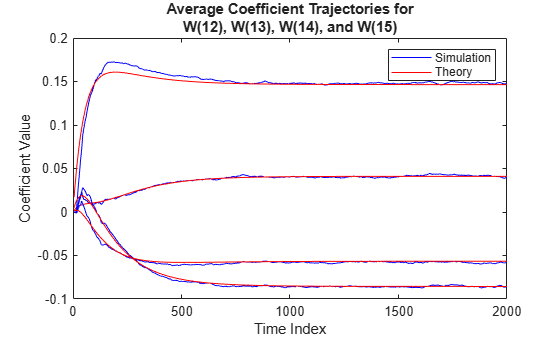In steady state, both the trajectories converge.

Sum of Squared Coefficient Errors

Compare the sum of the squared coefficient errors given by `msepred` and `msesim`. These values are given by the trace of the coefficient covariance matrix.

```semilogy(nn,traceKsim,nn,traceK,'r') title('Sum-of-Squared Coefficient Errors') axis([0 size(x,1) 0.0001 1]) legend('Simulation','Theory') xlabel('Time Index') ylabel('Squared Error Value')```The `maxstep` function computes the maximum step size of the adaptive filter. This step size keeps the filter stable at the maximum possible speed of convergence. Create the primary input signal, `x`, by passing a signed random signal to an IIR filter. Signal `x` contains 50 frames of 2000 samples each frame. Create an LMS filter with 32 taps and a step size of 0.1.

```x = zeros(2000,50); IIRFilter = dsp.IIRFilter('Numerator',sqrt(0.75),'Denominator',[1 -0.5]); for k = 1:size(x,2) x(:,k) = IIRFilter(sign(randn(size(x,1),1))); end mu = 0.1; LMSFilter = dsp.LMSFilter('Length',32,'StepSize',mu);```

Compute the maximum adaptation step size and the maximum step size in mean-squared sense using the `maxstep` function.

`[mumax,mumaxmse] = maxstep(LMSFilter,x)`
```mumax = 0.0625 ```
```mumaxmse = 0.0536 ```

System identification is the process of identifying the coefficients of an unknown system using an adaptive filter. The general overview of the process is shown in System Identification –– Using an Adaptive Filter to Identify an Unknown System. The main components involved are:

• The adaptive filter algorithm. In this example, set the `Method` property of `dsp.LMSFilter` to `'LMS'` to choose the LMS adaptive filter algorithm.

• An unknown system or process to adapt to. In this example, the filter designed by `fircband` is the unknown system.

• Appropriate input data to exercise the adaptation process. For the generic LMS model, these are the desired signal $\mathit{d}\left(\mathit{k}\right)$ and the input signal $\mathit{x}\left(\mathit{k}\right)$.

The objective of the adaptive filter is to minimize the error signal between the output of the adaptive filter $\mathit{y}\left(\mathit{k}\right)$ and the output of the unknown system (or the system to be identified) $\mathit{d}\left(\mathit{k}\right)$. Once the error signal is minimized, the adapted filter resembles the unknown system. The coefficients of both the filters match closely.

Note: If you are using R2016a or an earlier release, replace each call to the object with the equivalent step syntax. For example, `obj(x)` becomes `step(obj,x)`.

Unknown System

Create a `dsp.FIRFilter` object that represents the system to be identified. Use the `fircband` function to design the filter coefficients. The designed filter is a lowpass filter constrained to 0.2 ripple in the stopband.

```filt = dsp.FIRFilter; filt.Numerator = fircband(12,[0 0.4 0.5 1],[1 1 0 0],[1 0.2],... {'w' 'c'});```

Pass the signal `x` to the FIR filter. The desired signal `d` is the sum of the output of the unknown system (FIR filter) and an additive noise signal `n`.

```x = 0.1*randn(250,1); n = 0.01*randn(250,1); d = filt(x) + n;```

With the unknown filter designed and the desired signal in place, create and apply the adaptive LMS filter object to identify the unknown filter.

Preparing the adaptive filter object requires starting values for estimates of the filter coefficients and the LMS step size (`mu`). You can start with some set of nonzero values as estimates for the filter coefficients. This example uses zeros for the 13 initial filter weights. Set the `InitialConditions` property of `dsp.LMSFilter` to the desired initial values of the filter weights. For the step size, 0.8 is a good compromise between being large enough to converge well within 250 iterations (250 input sample points) and small enough to create an accurate estimate of the unknown filter.

Create a `dsp.LMSFilter` object to represent an adaptive filter that uses the LMS adaptive algorithm. Set the length of the adaptive filter to 13 taps and the step size to 0.8.

```mu = 0.8; lms = dsp.LMSFilter(13,'StepSize',mu)```
```lms = dsp.LMSFilter with properties: Method: 'LMS' Length: 13 StepSizeSource: 'Property' StepSize: 0.8000 LeakageFactor: 1 InitialConditions: 0 AdaptInputPort: false WeightsResetInputPort: false WeightsOutput: 'Last' Show all properties ```

Pass the primary input signal `x` and the desired signal `d` to the LMS filter. Run the adaptive filter to determine the unknown system. The output `y` of the adaptive filter is the signal converged to the desired signal d thereby minimizing the error `e `between the two signals.

Plot the results. The output signal does not match the desired signal as expected, making the error between the two nontrivial.

```[y,e,w] = lms(x,d); plot(1:250, [d,y,e]) title('System Identification of an FIR filter') legend('Desired','Output','Error') xlabel('Time index') ylabel('Signal value')```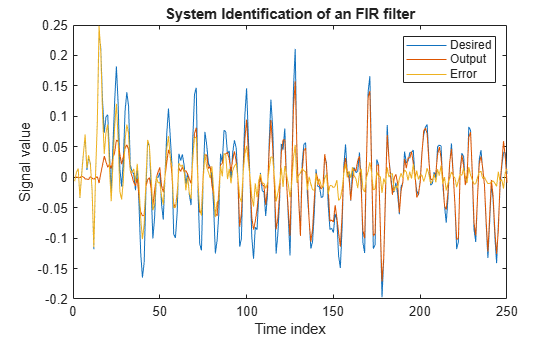Compare the Weights

The weights vector `w` represents the coefficients of the LMS filter that is adapted to resemble the unknown system (FIR filter). To confirm the convergence, compare the numerator of the FIR filter and the estimated weights of the adaptive filter.

The estimated filter weights do not closely match the actual filter weights, confirming the results seen in the previous signal plot.

```stem([(filt.Numerator).' w]) title('System Identification by Adaptive LMS Algorithm') legend('Actual filter weights','Estimated filter weights',... 'Location','NorthEast')```Changing the Step Size

As an experiment, change the step size to 0.2. Repeating the example with `mu = 0.2` results in the following stem plot. The filters do not converge, and the estimated weights are not good approxmations of the actual weights.

```mu = 0.2; lms = dsp.LMSFilter(13,'StepSize',mu); [~,~,w] = lms(x,d); stem([(filt.Numerator).' w]) title('System Identification by Adaptive LMS Algorithm') legend('Actual filter weights','Estimated filter weights',... 'Location','NorthEast')```Increase the Number of Data Samples

Increase the frame size of the desired signal. Even though this increases the computation involved, the LMS algorithm now has more data that can be used for adaptation. With 1000 samples of signal data and a step size of 0.2, the coefficients are aligned closer than before, indicating an improved convergence.

```release(filt); x = 0.1*randn(1000,1); n = 0.01*randn(1000,1); d = filt(x) + n; [y,e,w] = lms(x,d); stem([(filt.Numerator).' w]) title('System Identification by Adaptive LMS Algorithm') legend('Actual filter weights','Estimated filter weights',... 'Location','NorthEast')```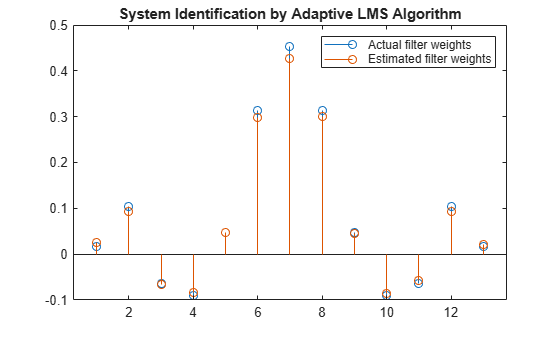Increase the number of data samples further by inputting the data through iterations. Run the algorithm on 4000 samples of data, passed to the LMS algorithm in batches of 1000 samples over 4 iterations.

Compare the filter weights. The weights of the LMS filter match the weights of the FIR filter very closely, indicating a good convergence.

```release(filt); n = 0.01*randn(1000,1); for index = 1:4 x = 0.1*randn(1000,1); d = filt(x) + n; [y,e,w] = lms(x,d); end stem([(filt.Numerator).' w]) title('System Identification by Adaptive LMS Algorithm') legend('Actual filter weights','Estimated filter weights',... 'Location','NorthEast')```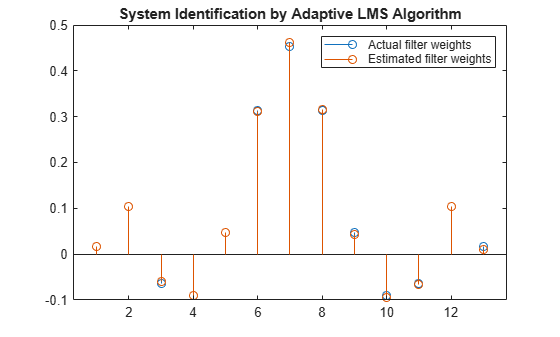The output signal matches the desired signal very closely, making the error between the two close to zero.

```plot(1:1000, [d,y,e]) title('System Identification of an FIR filter') legend('Desired','Output','Error') xlabel('Time index') ylabel('Signal value')```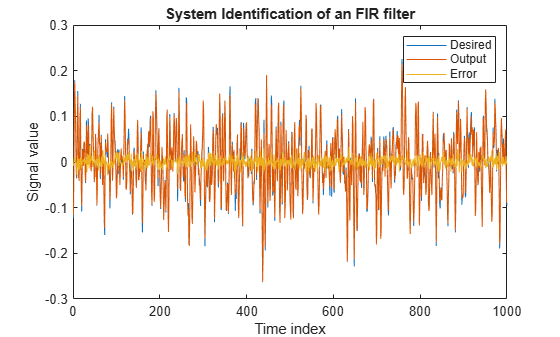To improve the convergence performance of the LMS algorithm, the normalized variant (NLMS) uses an adaptive step size based on the signal power. As the input signal power changes, the algorithm calculates the input power and adjusts the step size to maintain an appropriate value. The step size changes with time, and as a result, the normalized algorithm converges faster with fewer samples in many cases. For input signals that change slowly over time, the normalized LMS algorithm can be a more efficient LMS approach.

For an example using the LMS approach, see System Identification of FIR Filter Using LMS Algorithm.

Note: If you are using R2016a or an earlier release, replace each call to the object with the equivalent step syntax. For example, `obj(x)` becomes `step(obj,x)`.

Unknown System

Create a `dsp.FIRFilter` object that represents the system to be identified. Use the `fircband` function to design the filter coefficients. The designed filter is a lowpass filter constrained to 0.2 ripple in the stopband.

```filt = dsp.FIRFilter; filt.Numerator = fircband(12,[0 0.4 0.5 1],[1 1 0 0],[1 0.2],... {'w' 'c'});```

Pass the signal `x` to the FIR filter. The desired signal `d` is the sum of the output of the unknown system (FIR filter) and an additive noise signal `n`.

```x = 0.1*randn(1000,1); n = 0.001*randn(1000,1); d = filt(x) + n;```

To use the normalized LMS algorithm variation, set the `Method` property on the `dsp.LMSFilter` to `'Normalized LMS'`. Set the length of the adaptive filter to 13 taps and the step size to 0.2.

```mu = 0.2; lms = dsp.LMSFilter(13,'StepSize',mu,'Method',... 'Normalized LMS');```

Pass the primary input signal `x` and the desired signal `d` to the LMS filter.

`[y,e,w] = lms(x,d);`

The output `y` of the adaptive filter is the signal converged to the desired signal d thereby minimizing the error `e` between the two signals.

```plot(1:1000, [d,y,e]) title('System Identification by Normalized LMS Algorithm') legend('Desired','Output','Error') xlabel('Time index') ylabel('Signal value')```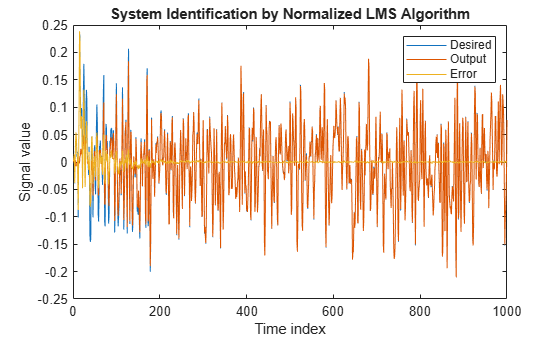Compare the Adapted Filter to the Unknown System

The weights vector w represents the coefficients of the LMS filter that is adapted to resemble the unknown system (FIR filter). To confirm the convergence, compare the numerator of the FIR filter and the estimated weights of the adaptive filter.

```stem([(filt.Numerator).' w]) title('System Identification by Normalized LMS Algorithm') legend('Actual filter weights','Estimated filter weights',... 'Location','NorthEast')```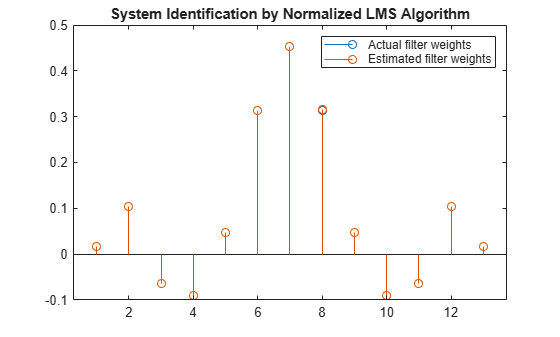An adaptive filter adapts its filter coefficients to match the coefficients of an unknown system. The objective is to minimize the error signal between the output of the unknown system and the output of the adaptive filter. When these two outputs converge and match closely for the same input, the coefficients are said to match closely. The adaptive filter at this state resembles the unknown system. This example compares the rate at which this convergence happens for the normalized LMS (NLMS) algorithm and the LMS algorithm with no normalization.

Unknown System

Create a `dsp.FIRFilter` that represents the unknown system. Pass the signal `x` as an input to the unknown system. The desired signal `d` is the sum of the output of the unknown system (FIR filter) and an additive noise signal `n`.

```filt = dsp.FIRFilter; filt.Numerator = fircband(12,[0 0.4 0.5 1],[1 1 0 0],[1 0.2],... {'w' 'c'}); x = 0.1*randn(1000,1); n = 0.001*randn(1000,1); d = filt(x) + n;```

Create two `dsp.LMSFilter` objects, with one set to the LMS algorithm, and the other set to the normalized LMS algorithm. Choose an adaptation step size of 0.2 and set the length of the adaptive filter to 13 taps.

```mu = 0.2; lms_nonnormalized = dsp.LMSFilter(13,'StepSize',mu,... 'Method','LMS'); lms_normalized = dsp.LMSFilter(13,'StepSize',mu,... 'Method','Normalized LMS');```

Pass the primary input signal `x` and the desired signal `d` to both the variations of the LMS algorithm. The variables `e1 `and `e2` represent the error between the desired signal and the output of the normalized and nonnormalized filters, respecitvely.

```[~,e1,~] = lms_normalized(x,d); [~,e2,~] = lms_nonnormalized(x,d);```

Plot the error signals for both variations. The error signal for the NLMS variant converges to zero much faster than the error signal for the LMS variant. The normalized version adapts in far fewer iterations to a result almost as good as the nonnormalized version.

```plot([e1,e2]); title('Comparing the LMS and NLMS Conversion Performance'); legend('NLMS derived filter weights', ... 'LMS derived filter weights','Location', 'NorthEast'); xlabel('Time index') ylabel('Signal value')```Cancel additive noise, n, added to an unknown system using an LMS adaptive filter. The LMS filter adapts its coefficients until its transfer function matches the transfer function of the unknown system as closely as possible. The difference between the output of the adaptive filter and the output of the unknown system represents the error signal, `e`. Minimizing this error signal is the objective of the adaptive filter.

The unknown system and the LMS filter process the same input signal, `x`, and produce outputs `d` and `y`, respectively. If the coefficients of the adaptive filter match the coefficients of the unknown system, the error, `e`, in effect represents the additive noise.

Note: If you are using R2016a or an earlier release, replace each call to the object with the equivalent step syntax. For example, `obj(x)` becomes `step(obj,x)`.

Create a `dsp.FIRFilter` System object to represent the unknown system. Create a `dsp.LMSFilter` object and set the length to 11 taps and the step size to 0.05. Create a sine wave to represent the noise added to the unknown system. View the signals in a time scope.

```FrameSize = 100; NIter = 10; lmsfilt2 = dsp.LMSFilter('Length',11,'Method','Normalized LMS', ... 'StepSize',0.05); firfilt2 = dsp.FIRFilter('Numerator', fir1(10,[.5, .75])); sinewave = dsp.SineWave('Frequency',0.01, ... 'SampleRate',1,'SamplesPerFrame',FrameSize); scope = timescope('TimeUnits','Seconds',... 'YLimits',[-3 3],'BufferLength',2*FrameSize*NIter, ... 'ShowLegend',true,'ChannelNames', ... {'Noisy signal', 'Error signal'}); ```

Create a random input signal, x and pass the signal to the FIR filter. Add a sine wave to the output of the FIR filter to generate the noisy signal, d. The signal, d is the output of the unknown system. Pass the noisy signal and the primary input signal to the LMS filter. View the noisy signal and the error signal in the time scope.

```for k = 1:NIter x = randn(FrameSize,1); d = firfilt2(x) + sinewave(); [y,e,w] = lmsfilt2(x,d); scope([d,e]) end release(scope) ```The error signal, `e`, is the sinusoidal noise added to the unknown system. Minimizing the error signal minimizes the noise added to the system.

When the amount of computation required to derive an adaptive filter drives your development process, the sign-data variant of the LMS (SDLMS) algorithm might be a very good choice, as demonstrated in this example.

In the standard and normalized variations of the LMS adaptive filter, coefficients for the adapting filter arise from the mean square error between the desired signal and the output signal from the unknown system. The sign-data algorithm changes the mean square error calculation by using the sign of the input data to change the filter coefficients.

When the error is positive, the new coefficients are the previous coefficients plus the error multiplied by the step size µ. If the error is negative, the new coefficients are again the previous coefficients minus the error multiplied by µ — note the sign change.

When the input is zero, the new coefficients are the same as the previous set.

In vector form, the sign-data LMS algorithm is:

`$\mathit{w}\left(\mathit{k}+1\right)=\mathit{w}\left(\mathit{k}\right)+\mu \mathit{e}\left(\mathit{k}\right)\mathrm{sgn}\left(\mathit{x}\left(\mathit{k}\right)\right),$`

where

`$\mathrm{sgn}\left(\mathit{x}\left(\mathit{k}\right)\right)=\left\{\begin{array}{c}1,\text{\hspace{0.17em}}\mathit{x}\left(\mathit{k}\right)>0\\ 0,\text{\hspace{0.17em}}\mathit{x}\left(\mathit{k}\right)=0\\ -1,\text{\hspace{0.17em}}\mathit{x}\left(\mathit{k}\right)<0\end{array}$`

with vector $\mathit{w}$ containing the weights applied to the filter coefficients and vector $\mathit{x}$ containing the input data. The vector $\mathit{e}$ is the error between the desired signal and the filtered signal. The objective of the SDLMS algorithm is to minimize this error. Step size is represented by $\mu$.

With a smaller $\mu$, the correction to the filter weights gets smaller for each sample, and the SDLMS error falls more slowly. A larger $\mu$ changes the weights more for each step, so the error falls more rapidly, but the resulting error does not approach the ideal solution as closely. To ensure a good convergence rate and stability, select $\mu$ within the following practical bounds.

`$0<\mu <\frac{1}{\mathit{N}\left\{\mathrm{InputSignalPower}\right\}},$`

where $\mathit{N}$ is the number of samples in the signal. Also, define $\mu$ as a power of two for efficient computing.

Note: How you set the initial conditions of the sign-data algorithm profoundly influences the effectiveness of the adaptation process. Because the algorithm essentially quantizes the input signal, the algorithm can become unstable easily.

A series of large input values, coupled with the quantization process might result in the error growing beyond all bounds. Restrain the tendency of the sign-data algorithm to get out of control by choosing a small step size $\left(\mu \ll 1\right)$ and setting the initial conditions for the algorithm to nonzero positive and negative values.

In this noise cancellation example, set the `Method` property of `dsp.LMSFilter` to `'Sign-Data LMS'`. This example requires two input data sets:

For the signal, use a sine wave. Note that `signal` is a column vector of 1000 elements.

`signal = sin(2*pi*0.055*(0:1000-1)');`

Now, add correlated white noise to `signal`. To ensure that the noise is correlated, pass the noise through a lowpass FIR filter and then add the filtered noise to the signal.

```noise = randn(1000,1); filt = dsp.FIRFilter; filt.Numerator = fir1(11,0.4); fnoise = filt(noise); d = signal + fnoise;```

`fnoise` is the correlated noise and `d` is now the desired input to the sign-data algorithm.

To prepare the `dsp.LMSFilter` object for processing, set the initial conditions of the filter weights and `mu `(`StepSize`). As noted earlier in this section, the values you set for `coeffs` and `mu` determine whether the adaptive filter can remove the noise from the signal path.

In System Identification of FIR Filter Using LMS Algorithm, you constructed a default filter that sets the filter coefficients to zeros. In most cases that approach does not work for the sign-data algorithm. The closer you set your initial filter coefficients to the expected values, the more likely it is that the algorithm remains well behaved and converges to a filter solution that removes the noise effectively.

For this example, start with the coefficients used in the noise filter (`filt.Numerator`), and modify them slightly so the algorithm has to adapt.

```coeffs = (filt.Numerator).'-0.01; % Set the filter initial conditions. mu = 0.05; % Set the step size for algorithm updating.```

With the required input arguments for `dsp.LMSFilter` prepared, construct the LMS filter object, run the adaptation, and view the results.

```lms = dsp.LMSFilter(12,'Method','Sign-Data LMS',... 'StepSize',mu,'InitialConditions',coeffs); [~,e] = lms(noise,d); L = 200; plot(0:L-1,signal(1:L),0:L-1,e(1:L)); title('Noise Cancellation by the Sign-Data Algorithm'); legend('Actual signal','Result of noise cancellation',... 'Location','NorthEast'); xlabel('Time index') ylabel('Signal values')```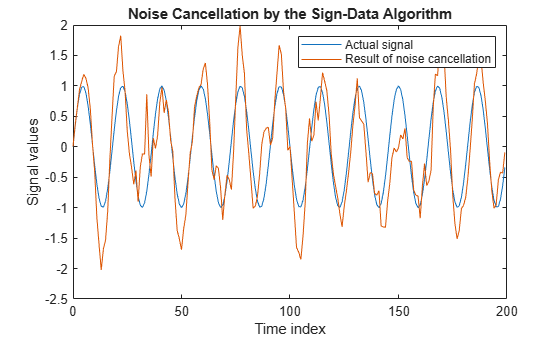When `dsp.LMSFilter` runs, it uses far fewer multiplication operations than either of the standard LMS algorithms. Also, performing the sign-data adaptation requires only multiplication by bit shifting when the step size is a power of two.

Although the performance of the sign-data algorithm as shown in this plot is quite good, the sign-data algorithm is much less stable than the standard LMS variations. In this noise cancellation example, the processed signal is a very good match to the input signal, but the algorithm could very easily grow without bound rather than achieve good performance.

Changing the weight initial conditions (`InitialConditions`) and `mu` (`StepSize`), or even the lowpass filter you used to create the correlated noise, can cause noise cancellation to fail.

In the standard and normalized variations of the LMS adaptive filter, the coefficients for the adapting filter arise from calculating the mean square error between the desired signal and the output signal from the unknown system, and applying the result to the current filter coefficients. The sign-error LMS (SELMS) algorithm replaces the mean square error calculation by using the sign of the error to modify the filter coefficients.

When the error is positive, the new coefficients are the previous coefficients plus the error multiplied by the step size $\mu$. If the error is negative, the new coefficients are the previous coefficients minus the error multiplied by $\mu$ — note the sign change. When the input is zero, the new coefficients are the same as the previous set.

In vector form, the sign-error LMS algorithm is:

$\mathit{w}\left(\mathit{k}+1\right)=\mathit{w}\left(\mathit{k}\right)+\mu \mathrm{sgn}\left(\mathit{e}\left(\mathit{k}\right)\right)\left(\mathit{x}\left(\mathit{k}\right)\right)$,

where

`$\mathrm{sgn}\left(\mathit{e}\left(\mathit{k}\right)\right)=\left\{\begin{array}{c}1,\text{\hspace{0.17em}}\mathit{e}\left(\mathit{k}\right)>0\\ 0,\text{\hspace{0.17em}}\mathit{e}\left(\mathit{k}\right)=0\\ -1,\text{\hspace{0.17em}}\mathit{e}\left(\mathit{k}\right)<0\end{array}$`

with vector $\mathit{w}$ containing the weights applied to the filter coefficients and vector $\mathit{x}$ containing the input data. The vector $\mathit{e}$ is the error between the desired signal and the filtered signal. The objective of the SELMS algorithm is to minimize this error.

With a smaller $\mu$, the correction to the filter weights gets smaller for each sample and the SELMS error falls more slowly. A larger $\mu$ changes the weights more for each step so the error falls more rapidly, but the resulting error does not approach the ideal solution as closely. To ensure a good convergence rate and stability, select $\mu$ within the following practical bounds.

`$0<\mu <\frac{1}{\mathit{N}\left\{\mathrm{InputSignalPower}\right\}}$`

where $\mathit{N}$ is the number of samples in the signal. Also, define $\mu$ as a power of two for efficient computation.

Note: How you set the initial conditions of the sign-error algorithm profoundly influences the effectiveness of the adaptation process. Because the algorithm essentially quantizes the error signal, the algorithm can become unstable easily.

A series of large error values, coupled with the quantization process might result in the error growing beyond all bounds. Restrain the tendency of the sign-error algorithm to become unstable by choosing a small step size $\left(\mu \ll 1\right)$ and setting the initial conditions for the algorithm to nonzero positive and negative values.

In this noise cancellation example, set the `Method` property of `dsp.LMSFilter` to `'Sign-Error LMS'`. This example requires two input data sets:

For the signal, use a sine wave. Note that `signal` is a column vector of 1000 elements.

`signal = sin(2*pi*0.055*(0:1000-1)');`

Now, add correlated white noise to `signal`. To ensure that the noise is correlated, pass the noise through a lowpass FIR filter and then add the filtered noise to the signal.

```noise = randn(1000,1); filt = dsp.FIRFilter; filt.Numerator = fir1(11,0.4); fnoise = filt(noise); d = signal + fnoise;```

`fnoise` is the correlated noise and `d` is now the desired input to the sign-error algorithm.

To prepare the `dsp.LMSFilter` object for processing, set the initial conditions of the filter weights (`InitialConditions`) and `mu` (`StepSize`). As noted earlier in this section, the values you set for `coeffs` and `mu` determine whether the adaptive filter can remove the noise from the signal path.

In System Identification of FIR Filter Using LMS Algorithm, you constructed a default filter that sets the filter coefficients to zeros. In most cases that approach does not work for the sign-error algorithm. The closer you set your initial filter coefficients to the expected values, the more likely it is that the algorithm remains well behaved and converges to a filter solution that removes the noise effectively.

For this example, start with the coefficients used in the noise filter (`filt.Numerator`) and modify them slightly so the algorithm has to adapt.

```coeffs = (filt.Numerator).'-0.01; % Set the filter initial conditions. mu = 0.05; % Set the step size for algorithm updating.```

With the required input arguments for `dsp.LMSFilter` prepared, run the adaptation and view the results.

```lms = dsp.LMSFilter(12,'Method','Sign-Error LMS',... 'StepSize',mu,'InitialConditions',coeffs); [~,e] = lms(noise,d); L = 200; plot(0:199,signal(1:200),0:199,e(1:200)); title('Noise cancellation performance by the sign-error LMS algorithm'); legend('Actual signal','Error after noise reduction',... 'Location','NorthEast') xlabel('Time index') ylabel('Signal value')```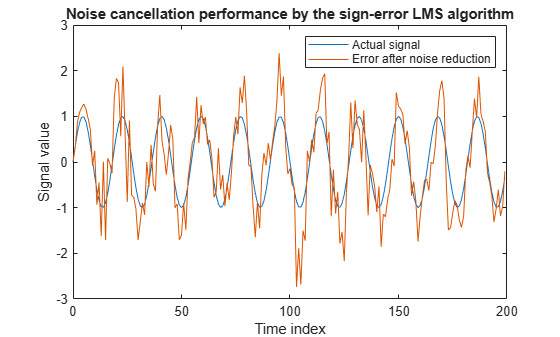When the sign-error LMS algorithm runs, it uses far fewer multiplication operations than either of the standard LMS algorithms. Also, performing the sign-error adaptation requires only bit shifting multiples when the step size is a power of two.

Although the performance of the sign-error algorithm as shown in this plot is quite good, the sign-error algorithm is much less stable than the standard LMS variations. In this noise cancellation example, the adapted signal is a very good match to the input signal, but the algorithm could very easily become unstable rather than achieve good performance.

Changing the weight initial conditions (`InitialConditions`) and `mu` (`StepSize`), or even the lowpass filter you used to create the correlated noise, can cause noise cancellation to fail and the algorithm to become useless.

The sign-sign LMS algorithm (SSLMS) replaces the mean square error calculation by using the sign of the input data to change the filter coefficients. When the error is positive, the new coefficients are the previous coefficients plus the error multiplied by the step size $\mu$. If the error is negative, the new coefficients are the previous coefficients minus the error multiplied by $\mu$ — note the sign change. When the input is zero, the new coefficients are the same as the previous set.

In essence, the algorithm quantizes both the error and the input by applying the sign operator to them.

In vector form, the sign-sign LMS algorithm is:

`$w\left(k+1\right)=w\left(k\right)+\mu \mathrm{sgn}\left(e\left(k\right)\right)\mathrm{sgn}\left(x\left(k\right)\right),$`

where

`$\mathrm{sgn}\left(\mathit{z}\left(\mathit{k}\right)\right)=\left\{\begin{array}{c}1,\text{\hspace{0.17em}}\mathit{z}\left(\mathit{k}\right)>0\\ 0,\text{\hspace{0.17em}}\mathit{z}\left(\mathit{k}\right)=0\\ -1,\text{\hspace{0.17em}}\mathit{z}\left(\mathit{k}\right)<0\end{array}$`

`$\mathit{z}\left(\mathit{k}\right)=\mathit{e}\left(\mathit{k}\right)\mathrm{sgn}\left(\mathit{x}\left(\mathit{k}\right)\right)$`

Vector $\mathit{w}$ contains the weights applied to the filter coefficients and vector $\mathit{x}$ contains the input data. The vector $\mathit{e}$ is the error between the desired signal and the filtered signal. The objective of the SSLMS algorithm is to minimize this error.

With a smaller $\mu$, the correction to the filter weights gets smaller for each sample and the SSLMS error falls more slowly. A larger $\mu$ changes the weights more for each step, so the error falls more rapidly, but the resulting error does not approach the ideal solution as closely. To ensure a good convergence rate and stability, select $\mu$ within the following practical bounds.

`$0<\mu <\frac{1}{\mathit{N}\left\{\mathrm{InputSignalPower}\right\}}$`

where $\mathit{N}$ is the number of samples in the signal. Also, define $\mu$ as a power of two for efficient computation

Note:

How you set the initial conditions of the sign-sign algorithm profoundly influences the effectiveness of the adaptation process. Because the algorithm essentially quantizes the input signal and the error signal, the algorithm can become unstable easily.

A series of large error values, coupled with the quantization process might result in the error growing beyond all bounds. Restrain the tendency of the sign-sign algorithm to become unstable by choosing a small step size $\left(\mu \ll 1\right)$ and setting the initial conditions for the algorithm to nonzero positive and negative values.

In this noise cancellation example, set the `Method` property of `dsp.LMSFilter` to `'Sign-Sign LMS'`. This example requires two input data sets:

For the signal, use a sine wave. Note that `signal` is a column vector of 1000 elements.

`signal = sin(2*pi*0.055*(0:1000-1)');`

Now, add correlated white noise to `signal`. To ensure that the noise is correlated, pass the noise through a lowpass FIR filter, then add the filtered noise to the signal.

```noise = randn(1000,1); filt = dsp.FIRFilter; filt.Numerator = fir1(11,0.4); fnoise = filt(noise); d = signal + fnoise;```

`fnoise` is the correlated noise and `d` is now the desired input to the sign-sign algorithm.

To prepare the `dsp.LMSFilter` object for processing, set the initial conditions of the filter weights (`InitialConditions`) and `mu` (`StepSize`). As noted earlier in this section, the values you set for `coeffs` and `mu` determine whether the adaptive filter can remove the noise from the signal path. In System Identification of FIR Filter Using LMS Algorithm, you constructed a default filter that sets the filter coefficients to zeros. Usually that approach does not work for the sign-sign algorithm.

The closer you set your initial filter coefficients to the expected values, the more likely it is that the algorithm remains well behaved and converges to a filter solution that removes the noise effectively. For this example, you start with the coefficients used in the noise filter (`filt.Numerator`), and modify them slightly so the algorithm has to adapt.

```coeffs = (filt.Numerator).' -0.01; % Set the filter initial conditions. mu = 0.05;```

With the required input arguments for `dsp.LMSFilter` prepared, run the adaptation and view the results.

```lms = dsp.LMSFilter(12,'Method','Sign-Sign LMS',... 'StepSize',mu,'InitialConditions',coeffs); [~,e] = lms(noise,d); L = 200; plot(0:199,signal(1:200),0:199,e(1:200)); title('Noise cancellation performance by the sign-sign LMS algorithm'); legend('Actual signal','Error after noise reduction',... 'Location','NorthEast') xlabel('Time index') ylabel('Signal value')```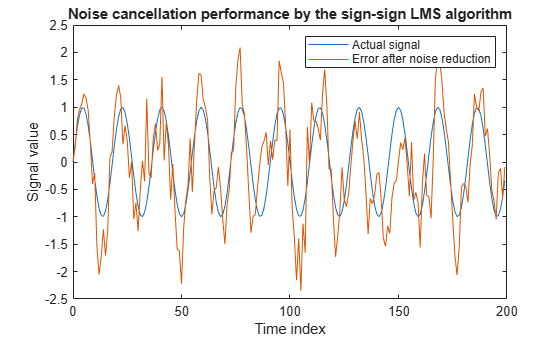When `dsp.LMSFilter` runs, it uses far fewer multiplication operations than either of the standard LMS algorithms. Also, performing the sign-sign adaptation requires only bit shifting multiples when the step size is a power of two.

Although the performance of the sign-sign algorithm as shown in this plot is quite good, the sign-sign algorithm is much less stable than the standard LMS variations. In this noise cancellation example, the adapted signal is a very good match to the input signal, but the algorithm could very easily become unstable rather than achieve good performance.

Changing the weight initial conditions (`InitialConditions`) and mu (`StepSize`), or even the lowpass filter you used to create the correlated noise, can cause noise cancellation to fail and the algorithm to become useless.

Note: This example runs only in R2017a or later. If you are using a release earlier than R2017a, the object does not output a full sample-by-sample history of filter weights. If you are using a release earlier than R2016b, replace each call to the function with the equivalent `step` syntax. For example, `myObject(x)` becomes `step(myObject,x)`.

Initialize the `dsp.LMSFilter` System object and set the `WeightsOutput` property to `'All'`. This setting enables the LMS filter to output a matrix of weights with dimensions `[FrameLength Length]`, corresponding to the full sample-by-sample history of weights for all `FrameLength` samples of input values.

```FrameSize = 15000; lmsfilt3 = dsp.LMSFilter('Length',63,'Method','LMS', ... 'StepSize',0.001,'LeakageFactor',0.99999, ... 'WeightsOutput','All'); % full Weights history w_actual = fir1(64,[0.5 0.75]); firfilt3 = dsp.FIRFilter('Numerator',w_actual); sinewave = dsp.SineWave('Frequency',0.01, ... 'SampleRate',1,'SamplesPerFrame',FrameSize); scope = timescope('TimeUnits','Seconds', ... 'YLimits',[-0.25 0.75],'BufferLength',2*FrameSize, ... 'ShowLegend',true,'ChannelNames', ... {'Coeff 33 Estimate','Coeff 34 Estimate','Coeff 35 Estimate', ... 'Coeff 33 Actual','Coeff 34 Actual','Coeff 35 Actual'}); ```

Run one frame and output the full adaptive weights history, `w`.

```x = randn(FrameSize,1); % Input signal d = firfilt3(x) + sinewave(); % Noise + Signal [~,~,w] = lmsfilt3(x,d); ```

Each row in `w` is a set of weights estimated for the respective input sample. Each column in `w` gives the complete history of a specific weight. Plot the actual weight and the entire history of the 33rd, 34th, and 35th weight. In the plot, you can see that the estimated weight output eventually converges with the actual weight as the adaptive filter receives input samples and continues to adapt.

```idxBeg = 33; idxEnd = 35; scope([w(:,idxBeg:idxEnd), repmat(w_actual(idxBeg:idxEnd),FrameSize,1)]) ```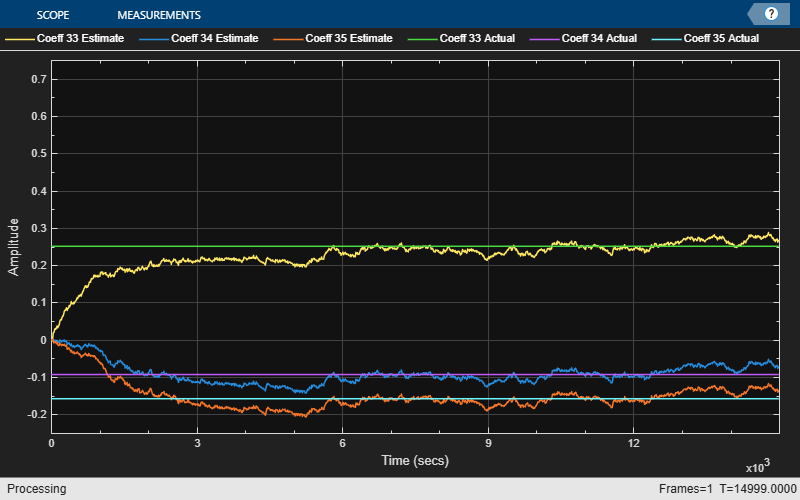## Algorithms

The LMS filter algorithm is defined by the following equations.

`$\begin{array}{c}y\left(n\right)={w}^{T}\left(n-1\right)u\left(n\right)\\ e\left(n\right)=d\left(n\right)-y\left(n\right)\\ w\left(n\right)=\alpha w\left(n-1\right)+f\left(u\left(n\right),e\left(n\right),\mu \right)\end{array}$`

The various LMS adaptive filter algorithms available in this System object are defined as:

• LMS –– Solves the Weiner-Hopf equation and finds the filter coefficients for an adaptive filter.

`$f\left(u\left(n\right),e\left(n\right),\mu \right)=\mu e\left(n\right){u}^{*}\left(n\right)$`

• Normalized LMS –– Normalized variation of the LMS algorithm.

`$f\left(u\left(n\right),e\left(n\right),\mu \right)=\mu e\left(n\right)\frac{{u}^{\ast }\left(n\right)}{\epsilon +{u}^{H}\left(n\right)u\left(n\right)}$`

In Normalized LMS, to overcome potential numerical instability in the update of the weights, a small positive constant, ε, has been added in the denominator. For double-precision floating-point input, ε is 2.2204460492503131e-016. For single-precision floating-point input, ε is 1.192092896e-07. For fixed-point input, ε is 0.

• Sign-Data LMS –– Correction to the filter weights at each iteration depends on the sign of the input u(n).

`$f\left(u\left(n\right),e\left(n\right),\mu \right)=\mu e\left(n\right)\text{sign}\left(u\left(n\right)\right)$`

where u(n) is real.

• Sign-Error LMS –– Correction applied to the current filter weights for each successive iteration depends on the sign of the error, e(n).

`$f\left(u\left(n\right),e\left(n\right),\mu \right)=\mu \text{sign}\left(e\left(n\right)\right){u}^{*}\left(n\right)$`

• Sign-Sign LMS –– Correction applied to the current filter weights for each successive iteration depends on both the sign of u(n) and the sign of e(n).

`$f\left(u\left(n\right),e\left(n\right),\mu \right)=\mu \text{sign}\left(e\left(n\right)\right)\text{sign}\left(u\left(n\right)\right)$`

where u(n) is real.

The variables are as follows:

VariableDescription

n

The current time index

u(n)

The vector of buffered input samples at step n

u*(n)

The complex conjugate of the vector of buffered input samples at step n

w(n)

The vector of filter weight estimates at step n

y(n)

The filtered output at step n

e(n)

The estimation error at step n

d(n)

The desired response at step n

µ

α

The leakage factor (0 < α ≤ 1)

ε

A constant that corrects any potential numerical instability that occurs during the update of weights.

 Hayes, M.H. Statistical Digital Signal Processing and Modeling. New York: John Wiley & Sons, 1996.

## SupportGet trial now